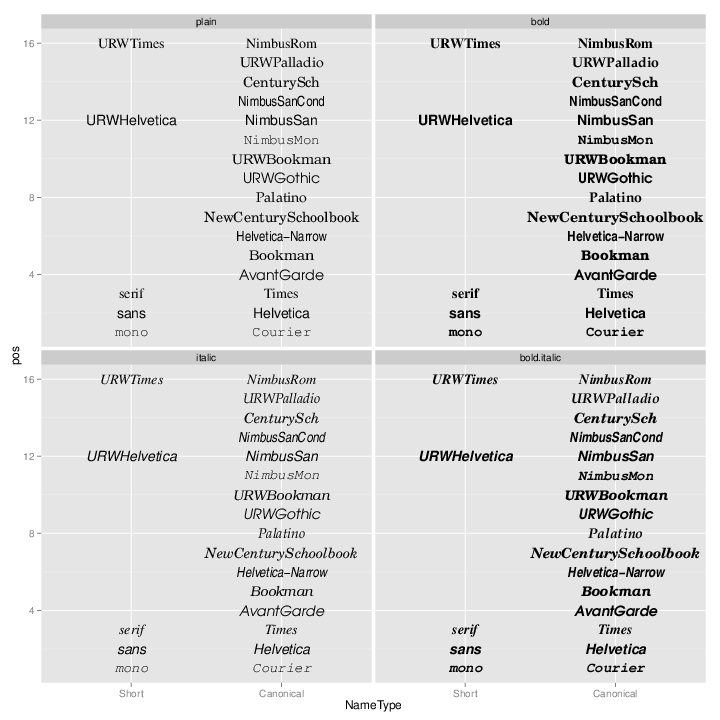## Problem

You want to use different fonts in your graphs.

## Solution

Update: Also see the extrafont package for much better support of fonts for PDF and Windows bitmap output.

Font support in R is generally not very good. It varies between systems, and between output formats.

### geom_text

With `geom_text` or `annotate` in ggplot2, you can set a number of properties of the text. `geom_text` is used to add text from the data frame, and `annotate` is used to add a single text element.

Name Default value
`size` 5
`family` `""` (sans)
`fontface` `plain`
`lineheight` 1.2
`angle` 0
`hjust` 0.5
`vjust` 0.5

Note that `size` is in mm, not points.

``````dat <- data.frame(
y = 1:3,
text = c("This is text", "Text with\nmultiple lines", "Some more text")
)

library(ggplot2)
p <- ggplot(dat, aes(x=1, y=y)) +
scale_y_continuous(limits=c(0.5, 3.5), breaks=NULL) +
scale_x_continuous(breaks=NULL)

p + geom_text(aes(label=text))

p + geom_text(aes(label=text), family="Times", fontface="italic", lineheight=.8) +
annotate(geom="text", x=1, y=1.5, label="Annotation text", colour="red",
size=7, family="Courier", fontface="bold", angle=30)
``````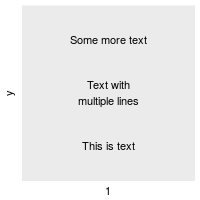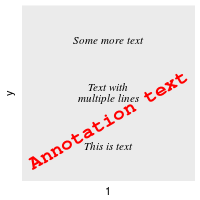### themes and element_text

When controlling elements such as the title, legend, axis labels, and so on, you use `element_text`, which has the same parameters, except that `size` is points (not mm), and instead of `fontface`, it uses `face`. The default value of `size` depends on the element; for example, titles are larger than tick labels.

``````p + geom_point() +
ggtitle("This is a Title") +
theme(plot.title=element_text(family="Times", face="bold", size=20))
``````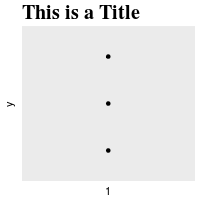### Table of fonts

You can use this code to generate a graphical table of fonts. Fonts have short names and canonical family names. You can use either one when specifying the family.

``````fonttable <- read.table(header=TRUE, sep=",", stringsAsFactors=FALSE,
text='
Short,Canonical
mono,Courier
sans,Helvetica
serif,Times
,AvantGarde
,Bookman
,Helvetica-Narrow
,NewCenturySchoolbook
,Palatino
,URWGothic
,URWBookman
,NimbusMon
URWHelvetica,NimbusSan
,NimbusSanCond
,CenturySch
URWTimes,NimbusRom
')

fonttable\$pos <- 1:nrow(fonttable)

library(reshape2)
fonttable <- melt(fonttable, id.vars="pos", measure.vars=c("Short","Canonical"),
variable.name="NameType", value.name="Font")

# Make a table of faces. Make sure factors are ordered correctly
facetable <- data.frame(Face = factor(c("plain","bold","italic","bold.italic"),
levels = c("plain","bold","italic","bold.italic")))

fullfonts <- merge(fonttable, facetable)

library(ggplot2)
pf <- ggplot(fullfonts, aes(x=NameType, y=pos)) +
geom_text(aes(label=Font, family=Font, fontface=Face)) +
facet_wrap(~ Face, ncol=2)
``````

To view it on screen:

``````pf
``````

What you see on the screen isn’t necessarily the same as what you get when you output to PNG or PDF. To see PNG output:

``````png('fonttable.png', width=720, height=720, res=72)
print(pf)
dev.off()
``````

Notice that on the system that generated this picture, most of the fonts (at the top) don’t really work. Only the basic fonts (at the bottom) work.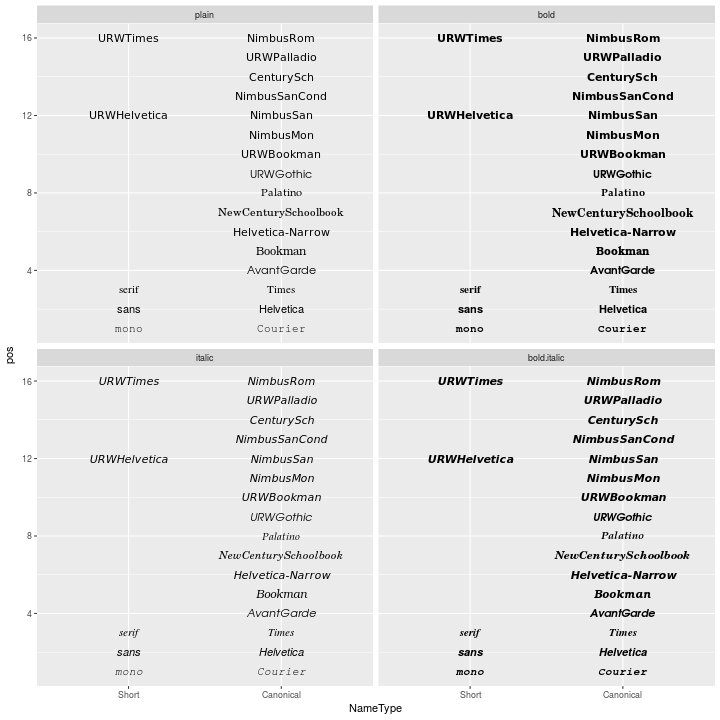And PDF output (the image below has been converted from PDF to PNG):

``````pdf('fonttable.pdf', width=10, height=10)
print(pf)
dev.off()

# Convert to PNG using GraphicsMagick:
# system("gm convert -resize 720x720 -background white fonttable.pdf fonttable-pdf.png")
``````

The PDF device has better font support than the PNG device. All the fonts work (though they’re not necessarily pretty):